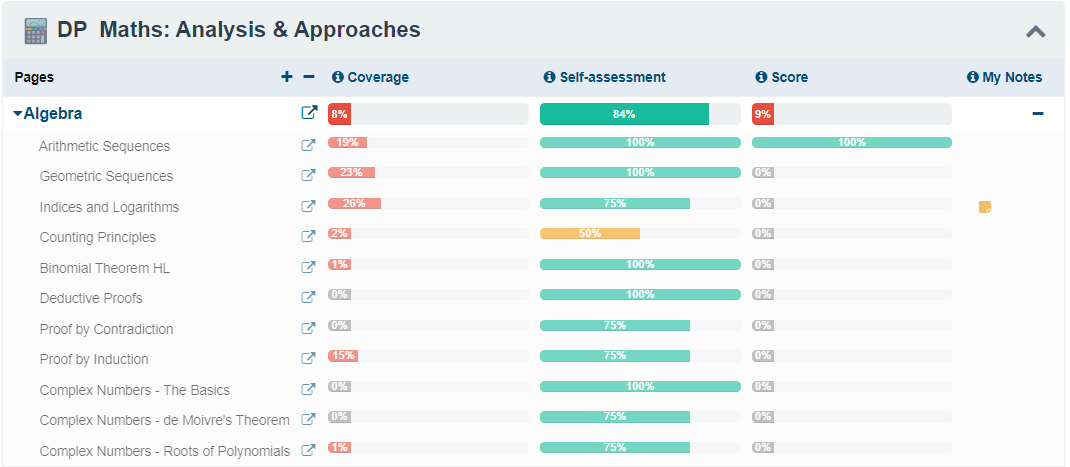# Start HereWelcome to the InThinking Mathematics Analysis and Approaches Revision Site! This site is designed to be your one-stop-virtual teacher for any help that you might need throughout the IB course. Whether you are trying to secure that all important grade 4, or wanting to change your 6s into 7s, this site has all you need:

#### Site Overview

See a quick overview of the site and some suggestions about how best to use it from here

• Video tutorials explaining key concepts.
• Concise revision notes providing you with reminders of all the key content you need
• Onscreen quizzes giving you instant feedback
• Exam style questions with hints, detailed solutions and explanation
• A huge questionbank designed to test your knowledge

You can track your own progress on the site#### Navigating the pages

Each of the topic pages is designed in the same way:

Key Concepts

Find out here exactly what you can expect to learn about on the page

Essentials

Had an explantion in class that you didn't quite understand? The short, high quality videos in this section will help you understand all the key concepts from each topic. Some are designed to explain concepts from the beginning and others are focussed on very specific skills and typical exam questions. All videos come with a set of notes that you can keep.

Here is an example video about understanding logarithms

Example - Understanding Logarithms

One of the important things about using logarithms is to be able to see them as indices, but just written in another way $$a^x=b \quad\Leftrightarrow \quad x=\log _{ a }{ b }$$

​Once you understand the conversion, then these questions become more about understanding indices.

In the following video, we will look at these 5 examples

$$1. \quad \log _{ 2 }{ 32 }=x \\ 2. \quad \log _{ 10}{ x }= 3\\ 3. \quad\ln{ 1 }=x \\ 4. \quad\log _{ 5 }{ \frac{1}{125} }=x \\ 5. \quad\log _{ 8}{ \frac{1}{4} } =x\\$$

#### Notes from the video

Summary

This section is like your own set of revision notes. Why not print them out so that you can add your own notes to them, then keep them together to make a complete revision guide for the whole course!

Here are an example set of notes on the topic Introducing Derivatives

Test Yourself

This section is all about getting practice. Before you try any exam-style questions, you will need a quick check to see if you have understood all the concepts from the page. These onscreen quizzes allow you to get just that. You can get instant feedback on your answers including advice about how to tackle the question and worked solutions.

Here is an example quiz on the topic of Arrangement in Counting Principles

Exam-style Questions

This is the section where you can practise questions just like you the ones that you will get in the exam. If you need some help getting started, there are hints. Each question has a full solution with explanation

Each of the questions is marked by difficulty levelEasyMediumHard

Here is an example question from the topic of Optimisation

Example - OptimisationThe diagram below shows the graph of the functions f(x) = sinx and g(x) = 2sinxA rectangle ABCD is placed in between the two functions as shown so that B and C lie on g , BC is parallel to the x axis and the local minima of the function f lies on AD.

Let NA = x

a) Find an expression for the height of the rectangle AB

b) Show that the area of the rectangle, A can be given by A = 4xcosx - 2x

c) Find $$\frac{dA}{dx}$$

d) Find the maximum value of the area of the rectangle.